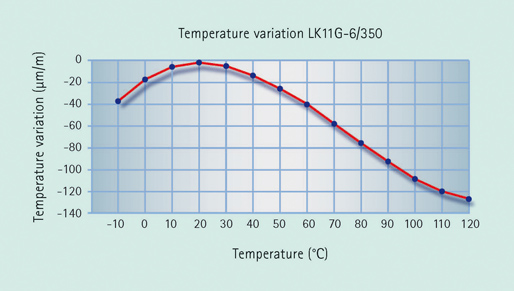Sensores de Força Baseados em Strain Gages Sensores de Força Baseados em Strain Gages | HBM

Force transducers based on strain gauges have a so-called spring element or loaded member where the forces to be measured are applied.

The spring element deforms and strain is produced on the surface. The task of the spring element is therefore to convert the forces to be measured into strain as reproducibly and linearly as possible. Many force transducer properties are defined by the selection of the spring element material and design.

The actual sensor element is the strain gauge (SG), which consists of an insulation layer, the so-called holder, with a measuring grid attached to it. Such strain gauges are bonded to the spring element at suitable points. In general, four strain gauges are used, installed so that two are stretched and two are compressed when force is applied.

These four SG are connected in a Wheatstone bridge circuit. As shown in the drawing below, the Wheatstone bridge is supplied with an excitation voltage. An output voltage then always occurs when the four resistances are different: e.g. when the resistance of the SG changes due to a strain.Working function of a force transducer based on strain gauges using the example of a ring torsion transducer (type: C18)

The output signal depends on the change in resistance of the SG and therefore directly on the applied force.

## Advantages of the strain gauge measurement principle

This principle has a proven track record and offers numerous advantages. The most important:

• If the electrical resistance of the SG changes in the same direction and to the same extent, an output voltage is not generated. This means that numerous parasitic influences, e.g. temperature dependence of the zero point, bending moment influences, lateral force effects, can be compensated for (see below)
• The measurement principle enables the production of force transducers with very high accuracies and comparably low costs.
• The nominal (rated) force of the transducer is only determined by the stiffness of the spring element. HBM has transducers available with nominal (rated) forces between 10 N and 5 MN.

The first point in particular is of significance. It shows that numerous unwanted influences can be compensated for by using a Wheatstone bridge circuit.
The influence of temperature on the zero point (TKZero) in particular can be compensated for to a very high level. Every strain gauge shows an output signal if the temperature changes, the so-called apparent strain.Figure 1: Output signal of an SG dependent on the temperature

## Compensation effect

The compensation effect results from the fact that all fourSG behave in the same way during temperature changes with regards to the direction and the amount of change in resistance. As two positive SG and two negative SG feed into the equation, there is essentially no output signal following a change in temperature.

The remaining, minute residual error can be corrected by special nickel elements that are connected to the Wheatstone bridge.

The addition of temperature-dependent resistances (also nickel elements) in the supply line compensates for the temperature dependence of the sensitivity(TCS). During temperature changes, the E-modulus of the material decreases which results in more strain under the same force application. In addition, the sensitivity of the SG is dependent on the temperature. The resistances in the supply line compensate for this in that the increase in resistance at higher temperature leads to a greater voltage drop. This reduces the voltage at the Wheatstone bridge and consequently the output signal decreases.

Linearity errors arise from the geometric ratios which change under load. Force transducers can be optimized to produce extremely good linearity through the clever selection of the spring element design and through precise positioning of the strain gauges.

Figure 2 shows a summary of compensation options. In addition to the TKzero and TCS influences described in detail above, it is also possible to compensate the linearity and to achieve the required sensitivity through adjustment.Figure 2: Connection of a force transducer with TKzero and TCS adjustment. Additional adjusting elements for sensitivity and linearity

The SG technology also permits the compensation of mechanical influences which should not be measured, e.g. bending moments or lateral forces.